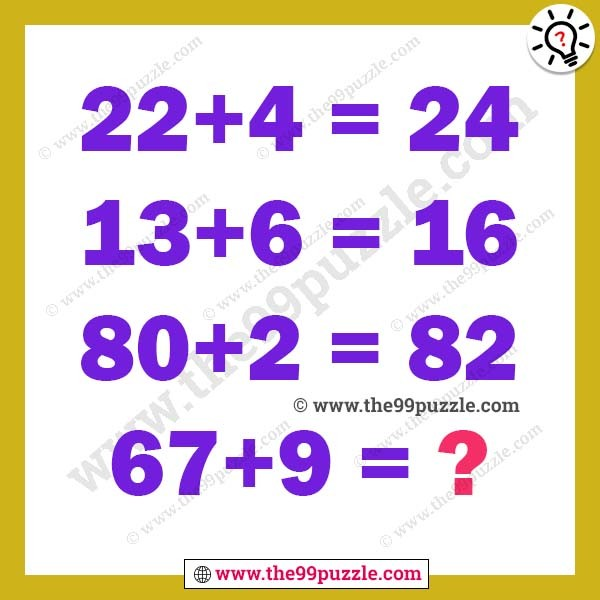# Tricky math brain riddle with answer – Puzz174

Tricky math brain riddle with answer. Logical maths is very fun. Here is the brain teaser in which there logical equations. Your challenge is to find the logical pattern in these equations and then find the value of the missing number. Simple math logic equation can solve both kids and adults all grade students. If you are intelligent people you can easily solve these puzzles.

22+4=24

13+6=16

80+2=82

67+9=?###### Explanation:

22+4 = 22+4−2 = 24

13+6 = 13+6−3 = 16

80+2 = 80+2−0 = 82

67+9 = 67+9−7 = 69